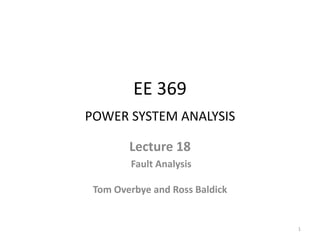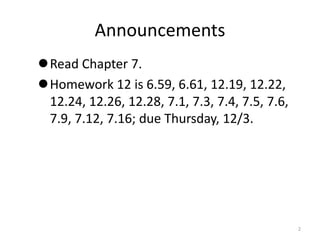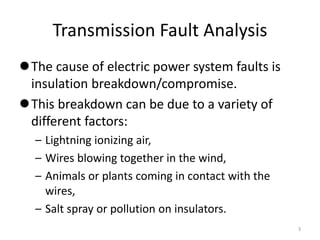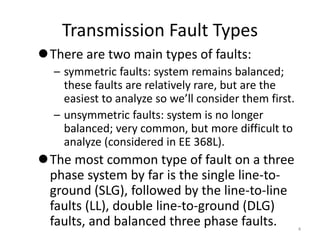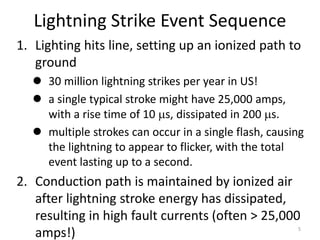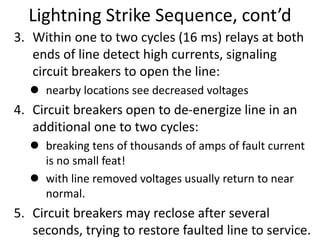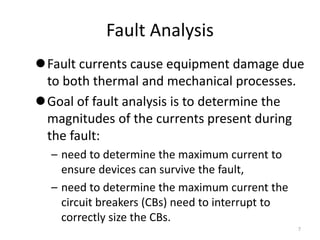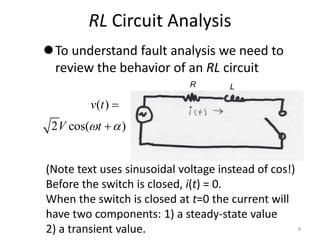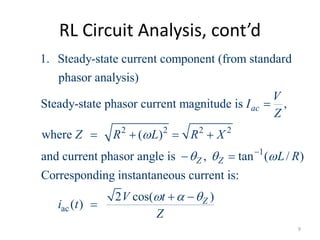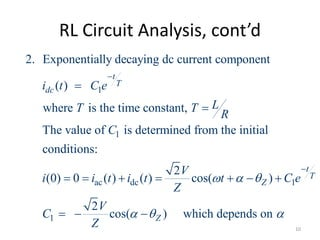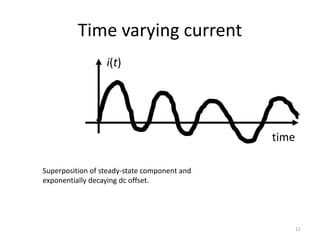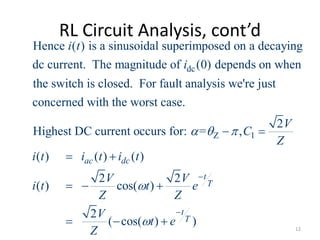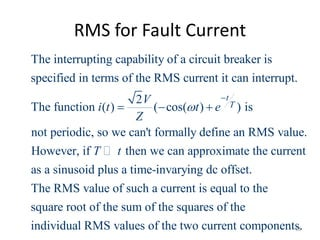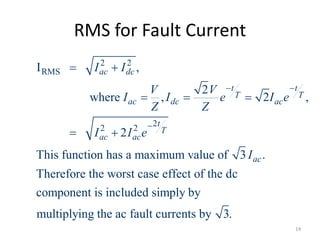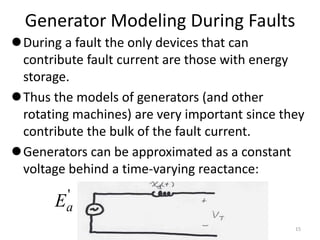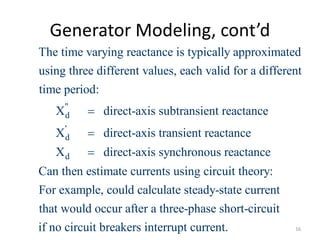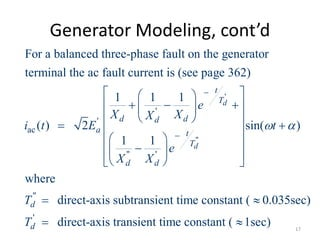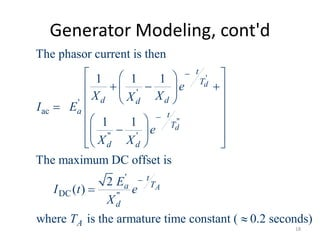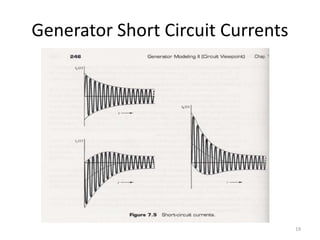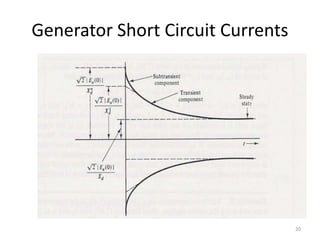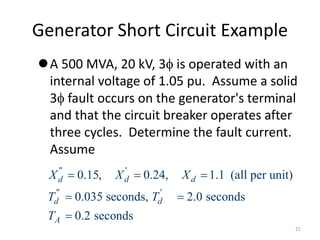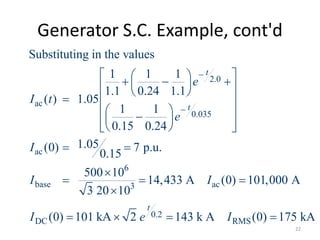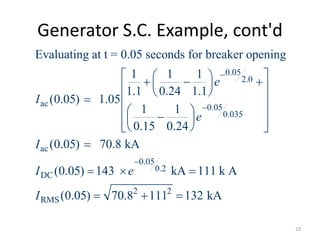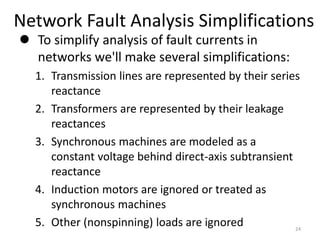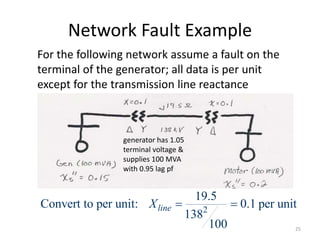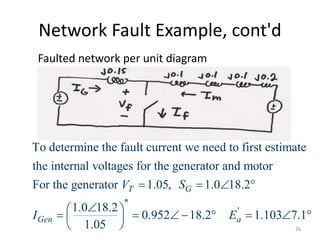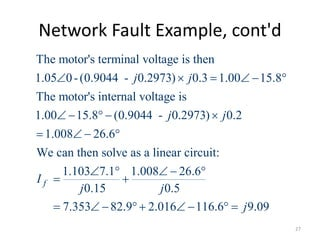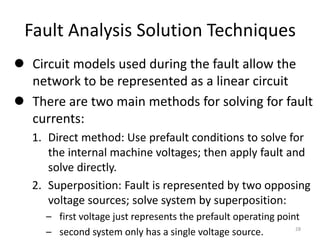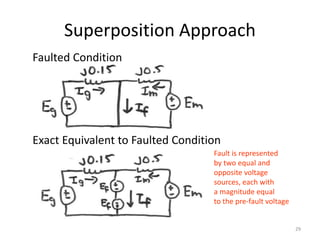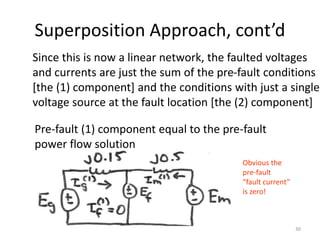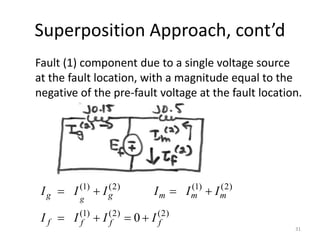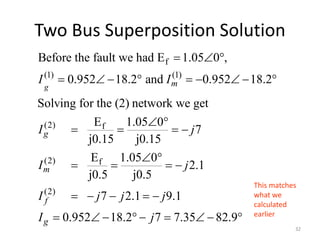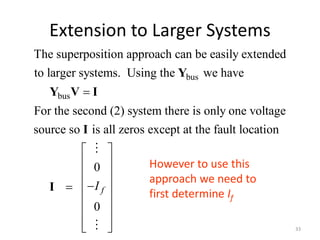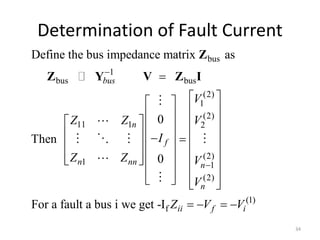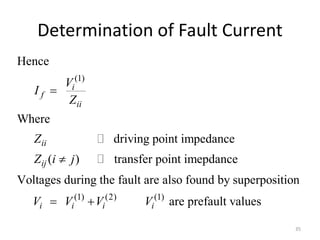1 of 35

### Lecture_18.ppt

1. EE 369 POWER SYSTEM ANALYSIS Lecture 18 Fault Analysis Tom Overbye and Ross Baldick 1
2. Announcements Read Chapter 7. Homework 12 is 6.59, 6.61, 12.19, 12.22, 12.24, 12.26, 12.28, 7.1, 7.3, 7.4, 7.5, 7.6, 7.9, 7.12, 7.16; due Thursday, 12/3. 2
3. Transmission Fault Analysis The cause of electric power system faults is insulation breakdown/compromise. This breakdown can be due to a variety of different factors: – Lightning ionizing air, – Wires blowing together in the wind, – Animals or plants coming in contact with the wires, – Salt spray or pollution on insulators. 3
4. Transmission Fault Types There are two main types of faults: – symmetric faults: system remains balanced; these faults are relatively rare, but are the easiest to analyze so we’ll consider them first. – unsymmetric faults: system is no longer balanced; very common, but more difficult to analyze (considered in EE 368L). The most common type of fault on a three phase system by far is the single line-to- ground (SLG), followed by the line-to-line faults (LL), double line-to-ground (DLG) faults, and balanced three phase faults. 4
5. Lightning Strike Event Sequence 1. Lighting hits line, setting up an ionized path to ground  30 million lightning strikes per year in US!  a single typical stroke might have 25,000 amps, with a rise time of 10 s, dissipated in 200 s.  multiple strokes can occur in a single flash, causing the lightning to appear to flicker, with the total event lasting up to a second. 2. Conduction path is maintained by ionized air after lightning stroke energy has dissipated, resulting in high fault currents (often > 25,000 amps!) 5
6. Lightning Strike Sequence, cont’d 3. Within one to two cycles (16 ms) relays at both ends of line detect high currents, signaling circuit breakers to open the line:  nearby locations see decreased voltages 4. Circuit breakers open to de-energize line in an additional one to two cycles:  breaking tens of thousands of amps of fault current is no small feat!  with line removed voltages usually return to near normal. 5. Circuit breakers may reclose after several seconds, trying to restore faulted line to service. 6
7. Fault Analysis Fault currents cause equipment damage due to both thermal and mechanical processes. Goal of fault analysis is to determine the magnitudes of the currents present during the fault: – need to determine the maximum current to ensure devices can survive the fault, – need to determine the maximum current the circuit breakers (CBs) need to interrupt to correctly size the CBs. 7
8. RL Circuit Analysis To understand fault analysis we need to review the behavior of an RL circuit ( ) 2 cos( ) v t V t     (Note text uses sinusoidal voltage instead of cos!) Before the switch is closed, i(t) = 0. When the switch is closed at t=0 the current will have two components: 1) a steady-state value 2) a transient value. R L 8
9. RL Circuit Analysis, cont’d 2 2 2 2 1 1. Steady-state current component (from standard phasor analysis) Steady-state phasor current magnitude is , where ( ) and current phasor angle is , tan ( / ) Corresponding in ac Z Z V I Z Z R L R X L R             ac stantaneous current is: 2 cos( ) ( ) Z V t i t Z       9
10. RL Circuit Analysis, cont’d 1 1 ac dc 1 1 2. Exponentially decaying dc current component ( ) where is the time constant, The value of is determined from the initial conditions: 2 (0) 0 ( ) ( ) cos( ) 2 t T dc t T Z i t C e L T T R C V i i t i t t C e Z V C Z                 cos( ) which depends on Z     10
11. Time varying current i(t) time Superposition of steady-state component and exponentially decaying dc offset. 11
12. RL Circuit Analysis, cont’d dc Hence ( ) is a sinusoidal superimposed on a decaying dc current. The magnitude of (0) depends on when the switch is closed. For fault analysis we're just concerned with the worst case. Highest DC c i t i Z 1 2 urrent occurs for: = , ( ) ( ) ( ) 2 2 ( ) cos( ) 2 ( cos( ) ) ac dc t T t T V C Z i t i t i t V V i t t e Z Z V t e Z                  12
13. RMS for Fault Current The interrupting capability of a circuit breaker is specified in terms of the RMS current it can interrupt. 2 The function ( ) ( cos( ) ) is not periodic, so we can't formally define an RMS value t T V i t t e Z      . However, if then we can approximate the current as a sinusoid plus a time-invarying dc offset. The RMS value of such a current is equal to the square root of the sum of the squares of the indivi T t dual RMS values of the two current components. 13
14. RMS for Fault Current 2 2 RMS 2 2 2 I , 2 where , 2 , 2 This function has a maximum value of 3 . Therefore the worst case effect of the dc component is included simply by mu ac dc t t T T ac dc ac t T ac ac ac I I V V I I e I e Z Z I I e I           ltiplying the ac fault currents by 3. 14
15. Generator Modeling During Faults During a fault the only devices that can contribute fault current are those with energy storage. Thus the models of generators (and other rotating machines) are very important since they contribute the bulk of the fault current. Generators can be approximated as a constant voltage behind a time-varying reactance: ' a E 15
16. Generator Modeling, cont’d " d ' d d The time varying reactance is typically approximated using three different values, each valid for a different time period: X direct-axis subtransient reactance X direct-axis transient reactance X dire    ct-axis synchronous reactance Can then estimate currents using circuit theory: For example, could calculate steady-state current that would occur after a three-phase short-circuit if no circuit breakers interrupt current. 16
17. Generator Modeling, cont’d ' " ' ' ac " ' " For a balanced three-phase fault on the generator terminal the ac fault current is (see page 362) 1 1 1 ( ) 2 sin( ) 1 1 where direct-axis su d d t T d d d a t T d d d e X X X i t E t e X X T                                      ' btransient time constant ( 0.035sec) direct-axis transient time constant ( 1sec) d T    17
18. Generator Modeling, cont'd ' " ' ' ac " ' ' DC " The phasor current is then 1 1 1 1 1 The maximum DC offset is 2 ( ) where is the armature time constant ( 0.2 seconds) d d A t T d d d a t T d d t T a d A e X X X I E e X X E I t e X T                                     18
19. Generator Short Circuit Currents 19
20. Generator Short Circuit Currents 20
21. Generator Short Circuit Example A 500 MVA, 20 kV, 3 is operated with an internal voltage of 1.05 pu. Assume a solid 3 fault occurs on the generator's terminal and that the circuit breaker operates after three cycles. Determine the fault current. Assume " ' " ' 0.15, 0.24, 1.1 (all per unit) 0.035 seconds, 2.0 seconds 0.2 seconds d d d d d A X X X T T T       21
22. Generator S.C. Example, cont'd 2.0 ac 0.035 ac 6 base ac 3 0.2 DC Substituting in the values 1 1 1 1.1 0.24 1.1 ( ) 1.05 1 1 0.15 0.24 1.05 (0) 7 p.u. 0.15 500 10 14,433 A (0) 101,000 A 3 20 10 (0) 101 kA 2 143 k t t t e I t e I I I I e                                          RMS A (0) 175 kA I  22
23. Generator S.C. Example, cont'd 0.05 2.0 ac 0.05 0.035 ac 0.05 0.2 DC RMS Evaluating at t = 0.05 seconds for breaker opening 1 1 1 1.1 0.24 1.1 (0.05) 1.05 1 1 0.15 0.24 (0.05) 70.8 kA (0.05) 143 kA 111 k A (0.05 e I e I I e I                                     2 2 ) 70.8 111 132 kA    23
24. Network Fault Analysis Simplifications  To simplify analysis of fault currents in networks we'll make several simplifications: 1. Transmission lines are represented by their series reactance 2. Transformers are represented by their leakage reactances 3. Synchronous machines are modeled as a constant voltage behind direct-axis subtransient reactance 4. Induction motors are ignored or treated as synchronous machines 5. Other (nonspinning) loads are ignored 24
25. Network Fault Example For the following network assume a fault on the terminal of the generator; all data is per unit except for the transmission line reactance 2 19.5 Convert to per unit: 0.1 per unit 138 100 line X   generator has 1.05 terminal voltage & supplies 100 MVA with 0.95 lag pf 25
26. Network Fault Example, cont'd Faulted network per unit diagram * ' To determine the fault current we need to first estimate the internal voltages for the generator and motor For the generator 1.05, 1.0 18.2 1.0 18.2 0.952 18.2 1.103 7.1 1.05 T G Gen a V S I E                    26
27. Network Fault Example, cont'd The motor's terminal voltage is then 1.05 0-(0.9044 - 0.2973) 0.3 1.00 15.8 The motor's internal voltage is 1.00 15.8 (0.9044 - 0.2973) 0.2 1.008 26.6 We can then solve as a linear circuit: 1 f j j j j I                 .103 7.1 1.008 26.6 0.15 0.5 7.353 82.9 2.016 116.6 9.09 j j j                27
28. Fault Analysis Solution Techniques  Circuit models used during the fault allow the network to be represented as a linear circuit  There are two main methods for solving for fault currents: 1. Direct method: Use prefault conditions to solve for the internal machine voltages; then apply fault and solve directly. 2. Superposition: Fault is represented by two opposing voltage sources; solve system by superposition: – first voltage just represents the prefault operating point – second system only has a single voltage source. 28
29. Superposition Approach Faulted Condition Exact Equivalent to Faulted Condition Fault is represented by two equal and opposite voltage sources, each with a magnitude equal to the pre-fault voltage 29
30. Superposition Approach, cont’d Since this is now a linear network, the faulted voltages and currents are just the sum of the pre-fault conditions [the (1) component] and the conditions with just a single voltage source at the fault location [the (2) component] Pre-fault (1) component equal to the pre-fault power flow solution Obvious the pre-fault “fault current” is zero! 30
31. Superposition Approach, cont’d Fault (1) component due to a single voltage source at the fault location, with a magnitude equal to the negative of the pre-fault voltage at the fault location. (1) (2) (1) (2) (1) (2) (2) 0 g g g m m m f f f f I I I I I I I I I I         31
32. Two Bus Superposition Solution f (1) (1) (2) f (2) f (2) Before the fault we had E 1.05 0 , 0.952 18.2 and 0.952 18.2 Solving for the (2) network we get E 1.05 0 7 j0.15 j0.15 E 1.05 0 2.1 j0.5 j0.5 7 2.1 9.1 0.952 g m g m f g I I I j I j I j j j I                                18.2 7 7.35 82.9 j        This matches what we calculated earlier 32
33. Extension to Larger Systems bus bus The superposition approach can be easily extended to larger systems. Using the we have For the second (2) system there is only one voltage source so is all zeros except at the fault loca  Y Y V I I tion 0 0 f I                   I However to use this approach we need to first determine If 33
34. Determination of Fault Current bus 1 bus bus (2) 1 (2) 11 1 2 (2) 1 1 (2) (1) f Define the bus impedance matrix as 0 Then 0 For a fault a bus i we get -I bus n f n nn n n ii f i V Z Z V I Z Z V V Z V V                                                      Z Z Y V Z I 34
35. Determination of Fault Current (1) (1) (2) (1) Hence Where driving point impedance ( ) transfer point imepdance Voltages during the fault are also found by superposition are prefault values i f ii ii ij i i i i V I Z Z Z i j V V V V     35